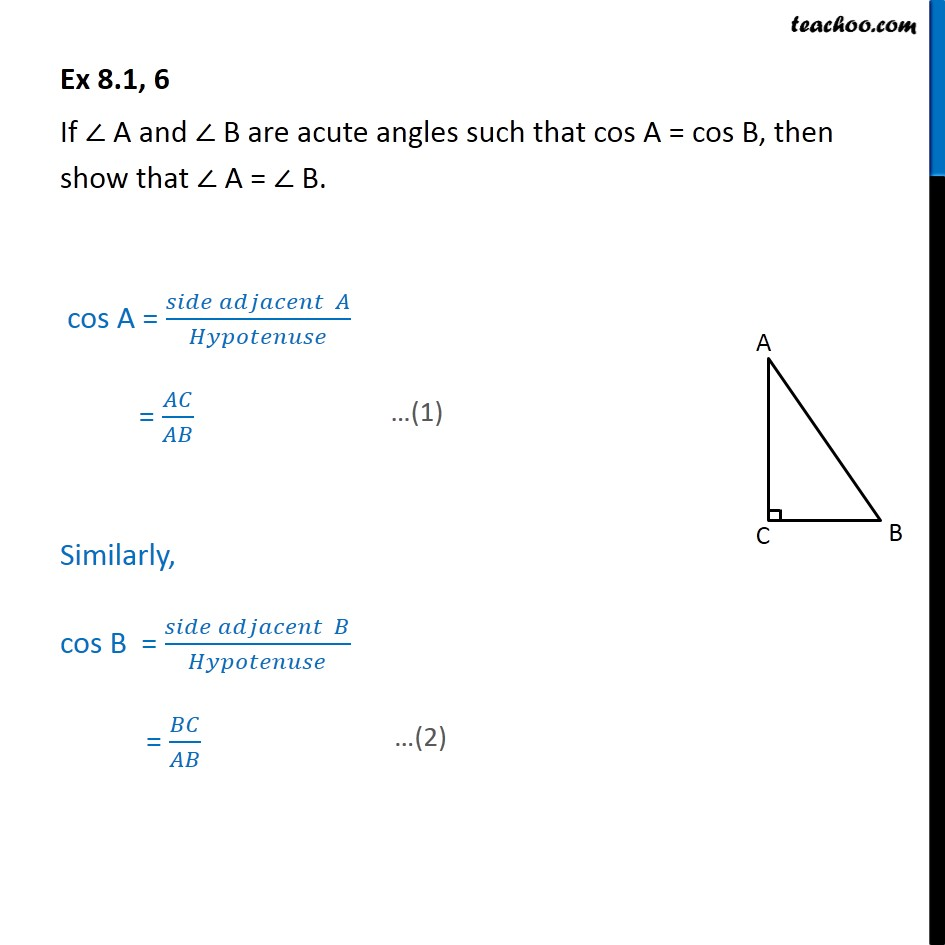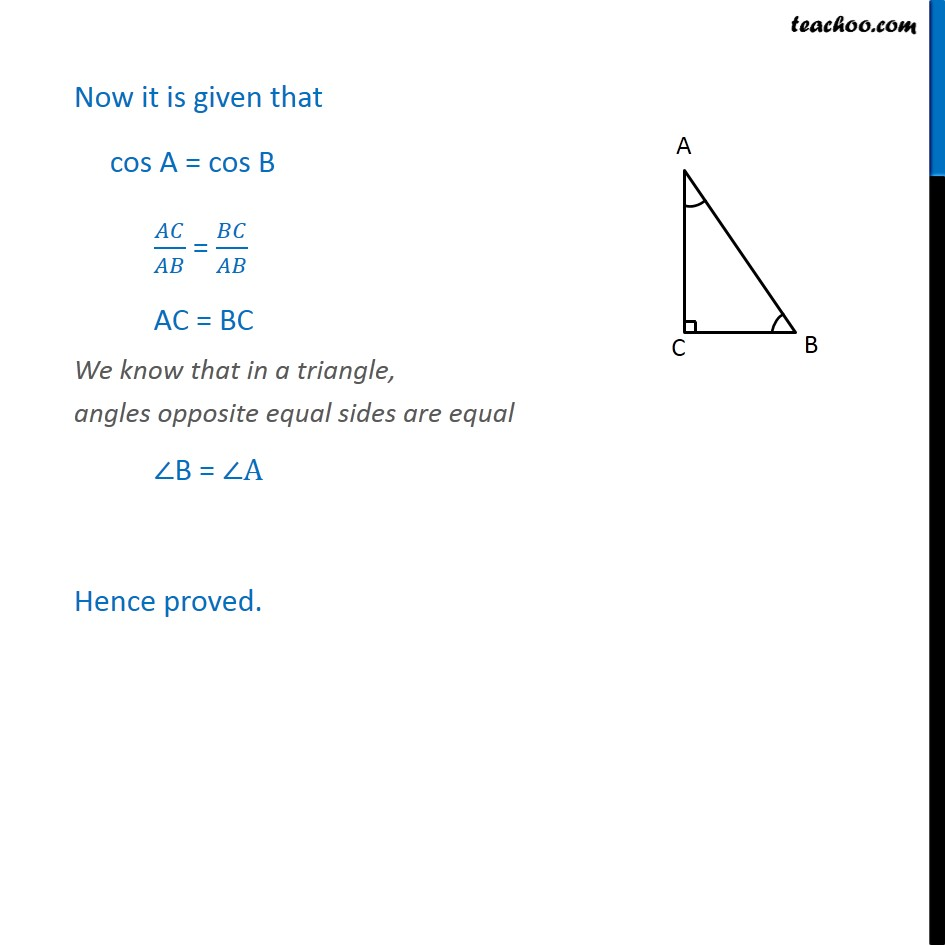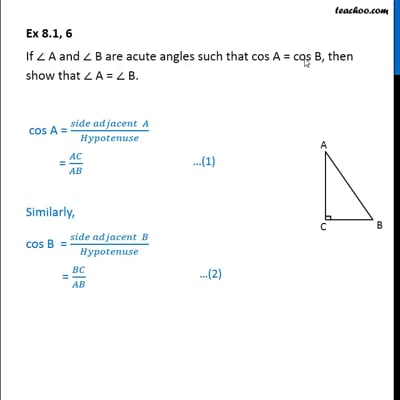Ex 8.1

Chapter 8 Class 10 Introduction to Trignometry (Term 1)
Serial order wiseThis video is only available for Teachoo black users

### Transcript

Ex 8.1, 6 If A and B are acute angles such that cos A = cos B, then show that A = B. cos A = (Side adjacent of angle A) / Hypotenuse = AC/AB Similarly, cos B = (Side adjacent of angle A) / Hypotenuse = BC/AB Now it is given that cos A = cos B AC/AB = BC/AB AC = BC We know that in a triangle, angles opposite equal sides are equal B = A Hence proved.